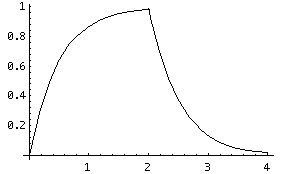# Capacitors and RC Circuits

The role of the capacitor in the electrical / fluid analogy is a little more complicated than that of the resistor. A capacitor is a device which allows us to store charge, and in this sense is analogous to the arterial walls, which store energy during pulsatile flow. However, the electrical analog to pulsatile flow is "alternating current" ("A.C."), and we would need to use calculus to adequately understand it. Fortunately, we can learn a great deal about electrical systems without getting into alternating current.

Capacitors store separated electric charges, and therefore energy. The "capacitance" of a capacitor is stated in terms of the amount of charge (Q) stored at a given voltage drop (across the capacitor):

C = Q / V.

The unit of capacitance is the "Farad", which is equal to a C / V. It is a very large unit; typical capacitances are measured in microfarads or picofarads. The canonical capacitor consists of a dielectric substance sandwiched between two parallel conducting plates. It has capacitance

C = e A / d,

where A is the plate surface area and d is the separation distance between the plates. In general, the capacitance of any capacitor depends only on the dielectric constant and the geometry of the capacitor. This is equivalent to the dependence of the energy of a charge configuration on the permittivity and the separation distances. The stored energy in a given capacitor at a given voltage is

C V 2 / 2.

Since the capacitor stores potential energy, there is a force trying to pull the plates together.

For a membrane, the capacitance C is measured per unit length. The membrane must be strong enough to resist the force resulting from the separation of charges on either side. Biological membranes typically have a capacitance of from 0.5 to 1.3 x 10 - 10 F / m. This means that they must store a charge on the order of 4 x 10 7 ions / m to create a rest potential. This corresponds to a stored energy of about 2 x 10 - 13 J / m. For a membrane thickness of 10 - 8 m, the force is on the order of 2 x 10 - 5 N / m.

Kirchoff's Laws also lead to rules for simplifying circuits with capacitors in series or parallel. Since capacitance is inversely proportional to voltage, capacitors in series are treated like resistors in parallel:

1/ C eq = 1 / C 1 + 1 / C 2.

Since capacitance is proportional to charge, which is proportional to current, the capacitance of a pair of parallel capacitors is simply the sum of their capacitances.

A circuit consisting of a battery, a switch, a resistor and a capacitor in a series loop is called an "RC" circuit. Kirchoff's voltage law for this circuit is

V = I R + Q / C.

When expressed purely in terms of the charge, this becomes

V = dQ/dt R + Q / C.

This is a "differential" equation, whose solution is an exponential function. When the switch is closed, the capacitor charges over time:

Q = Q f (1 - e - t / R C),

where Q is the charge at time t and Q f is the final charge on the capacitor. Note that Q never equals Q f , but as t gets extremely large, Q gets arbitrarily close to Q f . The product R C is called the "time constant", and is a characteristic quantity of the RC circuit. When t = R C, the capacitor has charged to a fraction (1 - 1 / e, about 63 %) of its final value. It is necessary to use a time constant, and not some sort of "terminal" time, since the process is asymptotic. Its value is an arbitrary choice; we "naturally" choose a value in terms of the exponential base (when the exponent is negative one).

When the capacitor is "fully" charged, the switch can be opened and (ideally) no charge will leak from the capacitor. If the battery is removed and the switch closed, the capacitor will then discharge over time:

Q = Q f e - t / R C.

In this scenario, the time constant represents the time that the capacitor has discharged to 1 / e (about 37 %) of its initial value. In the graph below, we see a charge and discharge with a time constant of 0.5:If this discussion seems a little pointless, we point out that every Node of Ranvier in your body acts like a little RC circuit. RC circuits are also widely used in commercial technology: your intermittant windshield wipers on your car are controlled by them.

The next section is about the cable model of the axon.

If you have stumbled on this page, and the equations look funny (or you just want to know where you are!), see the College Physics for Students of Biology and Chemistry home page.